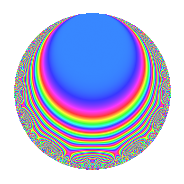Properties

 Label 350.2.aLevel 350 Weight 2 Character orbit a Rep. character $$\chi_{350}(1,\cdot)$$ Character field $$\Q$$ Dimension 10 Newforms 8 Sturm bound 120 Trace bound 3

Related objects

Defining parameters

 Level: $$N$$ = $$350 = 2 \cdot 5^{2} \cdot 7$$ Weight: $$k$$ = $$2$$ Character orbit: $$[\chi]$$ = 350.a (trivial) Character field: $$\Q$$ Newforms: $$8$$ Sturm bound: $$120$$ Trace bound: $$3$$ Distinguishing $$T_p$$: $$3$$, $$13$$

Dimensions

The following table gives the dimensions of various subspaces of $$M_{2}(\Gamma_0(350))$$.

Total New Old
Modular forms 72 10 62
Cusp forms 49 10 39
Eisenstein series 23 0 23

The following table gives the dimensions of the cuspidal new subspaces with specified eigenvalues for the Atkin-Lehner operators and the Fricke involution.

$$2$$$$5$$$$7$$FrickeDim.
$$+$$$$+$$$$+$$$$+$$$$1$$
$$+$$$$+$$$$-$$$$-$$$$2$$
$$+$$$$-$$$$+$$$$-$$$$2$$
$$-$$$$+$$$$+$$$$-$$$$1$$
$$-$$$$-$$$$+$$$$+$$$$1$$
$$-$$$$-$$$$-$$$$-$$$$3$$
Plus space$$+$$$$2$$
Minus space$$-$$$$8$$

Trace form

 $$10q$$ $$\mathstrut +\mathstrut 2q^{3}$$ $$\mathstrut +\mathstrut 10q^{4}$$ $$\mathstrut -\mathstrut 2q^{6}$$ $$\mathstrut +\mathstrut 18q^{9}$$ $$\mathstrut +\mathstrut O(q^{10})$$ $$10q$$ $$\mathstrut +\mathstrut 2q^{3}$$ $$\mathstrut +\mathstrut 10q^{4}$$ $$\mathstrut -\mathstrut 2q^{6}$$ $$\mathstrut +\mathstrut 18q^{9}$$ $$\mathstrut +\mathstrut 2q^{12}$$ $$\mathstrut +\mathstrut 10q^{13}$$ $$\mathstrut +\mathstrut 2q^{14}$$ $$\mathstrut +\mathstrut 10q^{16}$$ $$\mathstrut -\mathstrut 8q^{17}$$ $$\mathstrut +\mathstrut 4q^{18}$$ $$\mathstrut -\mathstrut 2q^{19}$$ $$\mathstrut +\mathstrut 6q^{21}$$ $$\mathstrut -\mathstrut 4q^{22}$$ $$\mathstrut -\mathstrut 2q^{24}$$ $$\mathstrut -\mathstrut 18q^{26}$$ $$\mathstrut -\mathstrut 4q^{27}$$ $$\mathstrut -\mathstrut 16q^{29}$$ $$\mathstrut +\mathstrut 4q^{31}$$ $$\mathstrut -\mathstrut 8q^{34}$$ $$\mathstrut +\mathstrut 18q^{36}$$ $$\mathstrut +\mathstrut 8q^{37}$$ $$\mathstrut +\mathstrut 2q^{38}$$ $$\mathstrut +\mathstrut 24q^{39}$$ $$\mathstrut -\mathstrut 12q^{41}$$ $$\mathstrut -\mathstrut 2q^{42}$$ $$\mathstrut -\mathstrut 12q^{43}$$ $$\mathstrut +\mathstrut 8q^{46}$$ $$\mathstrut +\mathstrut 4q^{47}$$ $$\mathstrut +\mathstrut 2q^{48}$$ $$\mathstrut +\mathstrut 10q^{49}$$ $$\mathstrut +\mathstrut 10q^{52}$$ $$\mathstrut -\mathstrut 4q^{53}$$ $$\mathstrut -\mathstrut 32q^{54}$$ $$\mathstrut +\mathstrut 2q^{56}$$ $$\mathstrut +\mathstrut 4q^{57}$$ $$\mathstrut -\mathstrut 12q^{58}$$ $$\mathstrut +\mathstrut 2q^{59}$$ $$\mathstrut -\mathstrut 6q^{61}$$ $$\mathstrut -\mathstrut 12q^{62}$$ $$\mathstrut -\mathstrut 4q^{63}$$ $$\mathstrut +\mathstrut 10q^{64}$$ $$\mathstrut -\mathstrut 12q^{66}$$ $$\mathstrut +\mathstrut 16q^{67}$$ $$\mathstrut -\mathstrut 8q^{68}$$ $$\mathstrut -\mathstrut 48q^{69}$$ $$\mathstrut -\mathstrut 48q^{71}$$ $$\mathstrut +\mathstrut 4q^{72}$$ $$\mathstrut -\mathstrut 4q^{73}$$ $$\mathstrut +\mathstrut 12q^{74}$$ $$\mathstrut -\mathstrut 2q^{76}$$ $$\mathstrut +\mathstrut 4q^{77}$$ $$\mathstrut +\mathstrut 8q^{78}$$ $$\mathstrut +\mathstrut 32q^{79}$$ $$\mathstrut -\mathstrut 18q^{81}$$ $$\mathstrut +\mathstrut 4q^{82}$$ $$\mathstrut -\mathstrut 2q^{83}$$ $$\mathstrut +\mathstrut 6q^{84}$$ $$\mathstrut -\mathstrut 20q^{86}$$ $$\mathstrut -\mathstrut 12q^{87}$$ $$\mathstrut -\mathstrut 4q^{88}$$ $$\mathstrut -\mathstrut 88q^{89}$$ $$\mathstrut +\mathstrut 10q^{91}$$ $$\mathstrut -\mathstrut 8q^{93}$$ $$\mathstrut -\mathstrut 4q^{94}$$ $$\mathstrut -\mathstrut 2q^{96}$$ $$\mathstrut +\mathstrut 8q^{97}$$ $$\mathstrut -\mathstrut 84q^{99}$$ $$\mathstrut +\mathstrut O(q^{100})$$

Decomposition of $$S_{2}^{\mathrm{new}}(\Gamma_0(350))$$ into irreducible Hecke orbits

Label Dim. $$A$$ Field CM Traces A-L signs $q$-expansion
$$a_2$$ $$a_3$$ $$a_5$$ $$a_7$$ 2 5 7
350.2.a.a $$1$$ $$2.795$$ $$\Q$$ None $$-1$$ $$-1$$ $$0$$ $$-1$$ $$+$$ $$+$$ $$+$$ $$q-q^{2}-q^{3}+q^{4}+q^{6}-q^{7}-q^{8}+\cdots$$
350.2.a.b $$1$$ $$2.795$$ $$\Q$$ None $$-1$$ $$0$$ $$0$$ $$1$$ $$+$$ $$+$$ $$-$$ $$q-q^{2}+q^{4}+q^{7}-q^{8}-3q^{9}+4q^{11}+\cdots$$
350.2.a.c $$1$$ $$2.795$$ $$\Q$$ None $$-1$$ $$3$$ $$0$$ $$1$$ $$+$$ $$+$$ $$-$$ $$q-q^{2}+3q^{3}+q^{4}-3q^{6}+q^{7}-q^{8}+\cdots$$
350.2.a.d $$1$$ $$2.795$$ $$\Q$$ None $$1$$ $$-3$$ $$0$$ $$-1$$ $$-$$ $$-$$ $$+$$ $$q+q^{2}-3q^{3}+q^{4}-3q^{6}-q^{7}+q^{8}+\cdots$$
350.2.a.e $$1$$ $$2.795$$ $$\Q$$ None $$1$$ $$1$$ $$0$$ $$1$$ $$-$$ $$-$$ $$-$$ $$q+q^{2}+q^{3}+q^{4}+q^{6}+q^{7}+q^{8}+\cdots$$
350.2.a.f $$1$$ $$2.795$$ $$\Q$$ None $$1$$ $$2$$ $$0$$ $$-1$$ $$-$$ $$+$$ $$+$$ $$q+q^{2}+2q^{3}+q^{4}+2q^{6}-q^{7}+q^{8}+\cdots$$
350.2.a.g $$2$$ $$2.795$$ $$\Q(\sqrt{6})$$ None $$-2$$ $$0$$ $$0$$ $$-2$$ $$+$$ $$-$$ $$+$$ $$q-q^{2}+\beta q^{3}+q^{4}-\beta q^{6}-q^{7}-q^{8}+\cdots$$
350.2.a.h $$2$$ $$2.795$$ $$\Q(\sqrt{6})$$ None $$2$$ $$0$$ $$0$$ $$2$$ $$-$$ $$-$$ $$-$$ $$q+q^{2}+\beta q^{3}+q^{4}+\beta q^{6}+q^{7}+q^{8}+\cdots$$

Decomposition of $$S_{2}^{\mathrm{old}}(\Gamma_0(350))$$ into lower level spaces

$$S_{2}^{\mathrm{old}}(\Gamma_0(350)) \cong$$ $$S_{2}^{\mathrm{new}}(\Gamma_0(14))$$$$^{\oplus 3}$$$$\oplus$$$$S_{2}^{\mathrm{new}}(\Gamma_0(35))$$$$^{\oplus 4}$$$$\oplus$$$$S_{2}^{\mathrm{new}}(\Gamma_0(50))$$$$^{\oplus 2}$$$$\oplus$$$$S_{2}^{\mathrm{new}}(\Gamma_0(70))$$$$^{\oplus 2}$$$$\oplus$$$$S_{2}^{\mathrm{new}}(\Gamma_0(175))$$$$^{\oplus 2}$$